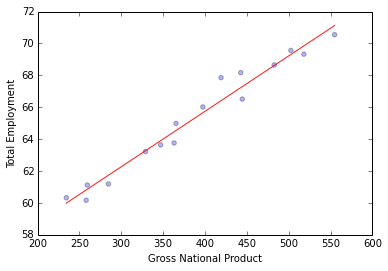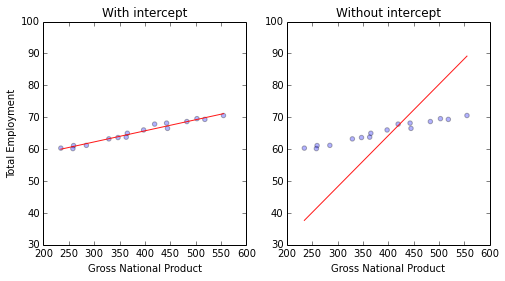# Ordinary Least Squares in Python¶

Linear Regression, also called Ordinary Least-Squares (OLS) Regression, is probably the most commonly used technique in Statistical Learning. It is also the oldest, dating back to the eighteenth century and the work of Carl Friedrich Gauss and Adrien-Marie Legendre. It is also one of the easier and more intuitive techniques to understand, and it provides a good basis for learning more advanced concepts and techniques. This posting explains how to perform linear regression using the statsmodels Python package, we will discuss the single variable case and defer multiple regression to a future post.

This is part of a series of blog posts to show how to do common statistical learning techniques in Python. We provide only a small amount of background on the concepts and techniques we cover, so if you'd like a more thorough explanation check out [Introduction to Statistical Learning](http://www-bcf.usc.edu/~gareth/ISL/) or sign up for the [free online course](http://online.stanford.edu/course/statistical-learning-winter-2014) by the authors here. If you are just here to learn how to do it in Python [skip](#skip-to-python) directly to the examples below.

## Statsmodels¶

Statsmodel is a Python library designed for more statistically-oriented approaches to data analysis, with an emphasis on econometric analyses. It integrates well with the pandas and numpy libraries we covered in a previous post. It also has built in support for many of the statistical tests to check the quality of the fit and a dedicated set of plotting functions to visualize and diagnose the fit. Scikit-learn also has support for linear regression, including many forms of regularized regression lacking in statsmodels, but it lacks the rich set of statistical tests and diagnostics that have been developed for linear models.

## Linear Regression and Ordinary Least Squares¶

Linear regression one of the simplest and most commonly used modeling techniques. It makes very strong assumptions about the relationship between the predictor variables (the X) and the response (the Y). It assumes that this relationship takes the form:

$y = \beta_0 + \beta_1 * x$

Ordinary Least Squares is the simplest and most common estimator in which the two $\beta$s are chosen to minimize the square of the distance between the predicted values and the actual values. Even though this model is quite rigid and often does not reflect the true relationship, this still remains a popular approach for several reasons. For one, it is computationally cheap to calculate the coefficients. It is also easier to interpret than more sophisticated models, and in situations where the goal is understanding a simple model in detail, rather than estimating the response well, they can provide insight into what the model captures. Finally, in situations where there is a lot of noise, it may be hard to find the true functional form, so a constrained model can perform quite well compared to a complex model which is more affected by noise.

The resulting model is represented as follows:

$\hat{y} = \hat{\beta}_0 + \hat{\beta}_1 * x$

Here the hats on the variables represent the fact that they are estimated from the data we have available. The $\beta$s are termed the parameters of the model or the coefficients. $\beta_0$ is called the constant term or the intercept.

## Ordinary Least Squares Using Statsmodels¶

The statsmodels package provides several different classes that provide different options for linear regression. Getting started with linear regression is quite straightforward with the OLS module.

In :
# load numpy and pandas for data manipulation
import numpy as np
import pandas as pd

# load statsmodels as alias sm
import statsmodels.api as sm

# load the longley dataset into a pandas data frame - first column (year) used as row labels

Out:
GNP.deflator GNP Unemployed Armed.Forces Population Year Employed
1947 83.0 234.289 235.6 159.0 107.608 1947 60.323
1948 88.5 259.426 232.5 145.6 108.632 1948 61.122
1949 88.2 258.054 368.2 161.6 109.773 1949 60.171
1950 89.5 284.599 335.1 165.0 110.929 1950 61.187
1951 96.2 328.975 209.9 309.9 112.075 1951 63.221

We will use the variable Total Derived Employment ('Employed') as our response y and Gross National Product ('GNP') as our predictor X.

We take the single response variable and store it separately. We also add a constant term so that we fit the intercept of our linear model.

In :
y = df.Employed  # response
X = df.GNP  # predictor

Out:
const GNP
1947 1 234.289
1948 1 259.426
1949 1 258.054
1950 1 284.599
1951 1 328.975

Now we perform the regression of the predictor on the response, using the sm.OLS class and and its initialization OLS(y, X) method. This method takes as an input two array-like objects: X and y. In general, X will either be a numpy array or a pandas data frame with shape (n, p) where n is the number of data points and p is the number of predictors. y is either a one-dimensional numpy array or a pandas series of length n.

In :
est = sm.OLS(y, X)


We then need to fit the model by calling the OLS object's fit() method. Ignore the warning about the kurtosis test if it appears, we have only 16 examples in our dataset and the test of the kurtosis is valid only if there are more than 20 examples.

In :
est = est.fit()
est.summary()

/usr/local/lib/python2.7/dist-packages/scipy/stats/stats.py:1276: UserWarning: kurtosistest only valid for n>=20 ... continuing anyway, n=16
int(n))

Out:
Dep. Variable: R-squared: Employed 0.967 OLS 0.965 Least Squares 415.1 Sat, 08 Feb 2014 8.36e-12 01:28:29 -14.904 16 33.81 14 35.35 1
coef std err t P>|t| [95.0% Conf. Int.] 51.8436 0.681 76.087 0.000 50.382 53.305 0.0348 0.002 20.374 0.000 0.031 0.038
 Omnibus: Durbin-Watson: 1.925 1.619 0.382 1.215 0.664 0.545 2.759 1660

After visualizing the relationship we will explain the summary. First, we need the coefficients of the fit.

In :
est.params

Out:
const    51.843590
GNP       0.034752
dtype: float64
In :
# Make sure that graphics appear inline in the iPython notebook
%pylab inline

# We pick 100 hundred points equally spaced from the min to the max
X_prime = np.linspace(X.GNP.min(), X.GNP.max(), 100)[:, np.newaxis]

# Now we calculate the predicted values
y_hat = est.predict(X_prime)

plt.scatter(X.GNP, y, alpha=0.3)  # Plot the raw data
plt.xlabel("Gross National Product")
plt.ylabel("Total Employment")
plt.plot(X_prime[:, 1], y_hat, 'r', alpha=0.9)  # Add the regression line, colored in red

Populating the interactive namespace from numpy and matplotlib

Out:
[<matplotlib.lines.Line2D at 0x4444350>]Statsmodels also provides a formulaic interface that will be familiar to users of R. Note that this requires the use of a different api to statsmodels, and the class is now called ols rather than OLS. The argument formula allows you to specify the response and the predictors using the column names of the input data frame data.

In :
# import formula api as alias smf
import statsmodels.formula.api as smf

# formula: response ~ predictors
est = smf.ols(formula='Employed ~ GNP', data=df).fit()
est.summary()

Out:
Dep. Variable: R-squared: Employed 0.967 OLS 0.965 Least Squares 415.1 Sat, 08 Feb 2014 8.36e-12 01:28:29 -14.904 16 33.81 14 35.35 1
coef std err t P>|t| [95.0% Conf. Int.] 51.8436 0.681 76.087 0.000 50.382 53.305 0.0348 0.002 20.374 0.000 0.031 0.038
 Omnibus: Durbin-Watson: 1.925 1.619 0.382 1.215 0.664 0.545 2.759 1660

This summary provides quite a lot of information about the fit. The pats of the table we think are the most important are bolded in the description below.

The left part of the first table provides basic information about the model fit:

Element Description
Dep. Variable Which variable is the response in the model
Model What model you are using in the fit
Method How the parameters of the model were calculated
No. Observations The number of observations (examples)
DF Residuals Degrees of freedom of the residuals. Number of observations - number of parameters
DF Model Number of parameters in the model (not including the constant term if present)

The right part of the first table shows the goodness of fit

Element Description
**R-squared** The [coefficient of determination](http://en.wikipedia.org/wiki/Coefficient_of_determination). A statistical measure of how well the regression line approximates the real data points
**Adj. R-squared** The above value adjusted based on the number of observations and the degrees-of-freedom of the residuals
F-statistic A measure how significant the fit is. The mean squared error of the model divided by the mean squared error of the residuals
Prob (F-statistic) The probability that you would get the above statistic, given the null hypothesis that they are unrelated
Log-likelihood The log of the likelihood function.
AIC The [Akaike Information Criterion](http://en.wikipedia.org/wiki/Akaike_information_criterion). Adjusts the log-likelihood based on the number of observations and the complexity of the model.
BIC The [Bayesian Information Criterion](http://en.wikipedia.org/wiki/Bayesian_information_criterion). Similar to the AIC, but has a higher penalty for models with more parameters.

The second table reports for each of the coefficients

Description
The name of the term in the model
coef The estimated value of the coefficient
std err The basic standard error of the estimate of the coefficient. More sophisticated errors are also available.
t The t-statistic value. This is a measure of how statistically significant the coefficient is.
P > |t| P-value that the null-hypothesis that the coefficient = 0 is true. If it is less than the confidence level, often 0.05, it indicates that there is a statistically significant relationship between the term and the response.
**[95.0% Conf. Interval]** The lower and upper values of the 95% confidence interval

Finally, there are several statistical tests to assess the distribution of the residuals

Element Description
Skewness A measure of the symmetry of the data about the mean. Normally-distributed errors should be symmetrically distributed about the mean (equal amounts above and below the line).
Kurtosis A measure of the shape of the distribution. Compares the amount of data close to the mean with those far away from the mean (in the tails).
Omnibus D'Angostino's test. It provides a combined statistical test for the presence of skewness and kurtosis.
Prob(Omnibus) The above statistic turned into a probability
Jarque-Bera A different test of the skewness and kurtosis
Prob (JB) The above statistic turned into a probability
Durbin-Watson A test for the presence of autocorrelation (that the errors are not independent.) Often important in time-series analysis
Cond. No A test for multicollinearity (if in a fit with multiple parameters, the parameters are related with each other).

As a final note, if you don't want to include a constant term in your model, you can exclude it using the minus operator.

In :
# Fit the no-intercept model
est_no_int = smf.ols(formula='Employed ~ GNP - 1', data=df).fit()

# We pick 100 hundred points equally spaced from the min to the max
X_prime_1 = pd.DataFrame({'GNP': np.linspace(X.GNP.min(), X.GNP.max(), 100)})

y_hat_int = est.predict(X_prime_1)
y_hat_no_int = est_no_int.predict(X_prime_1)

fig = plt.figure(figsize=(8,4))
splt = plt.subplot(121)

splt.scatter(X.GNP, y, alpha=0.3)  # Plot the raw data
plt.ylim(30, 100)  # Set the y-axis to be the same
plt.xlabel("Gross National Product")
plt.ylabel("Total Employment")
plt.title("With intercept")
splt.plot(X_prime[:, 1], y_hat_int, 'r', alpha=0.9)  # Add the regression line, colored in red

splt = plt.subplot(122)
splt.scatter(X.GNP, y, alpha=0.3)  # Plot the raw data
plt.xlabel("Gross National Product")
plt.title("Without intercept")
splt.plot(X_prime[:, 1], y_hat_no_int, 'r', alpha=0.9)  # Add the regression line, colored in red

Out:
[<matplotlib.lines.Line2D at 0x47eab50>]But notice that this may not be the best idea... :)

## Correlation and Causation¶

Clearly there is a relationship or correlation between GNP and total employment. So does that mean a change in GNP cause a change in total employment? Or does a change in total employment cause a change in GNP? This is a subject we will explore in the next post.